Escape Velocity Formula

The escape velocity is the minimum velocity required to leave a planet or moon. For a rocket or other object to leave a planet, it must overcome the pull of gravity. The formula for escape velocity contains a constant, G, which is called the “universal gravitational constant”. Its value is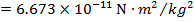. The unit for escape velocity is meters per second (m/s).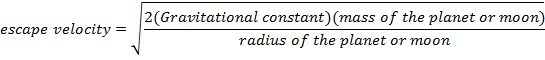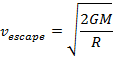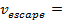escape velocity (m/s)

G = universal gravitational constant (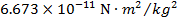)

M = mass of the planet or moon (kg)

R = radius of the planet or moon (m)

Escape Velocity Formula Questions:

1) The radius of Earth is 6.38×10m, and the mass of the Earth is 5.98×1024 kg. What is the escape velocity from Earth?

Answer: The escape velocity from Earth can be found using the formula: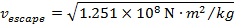11184 m/s

The escape velocity from Earth is 11 184 m/s, or approximately 11.2km/s.

2) To leave the moon, the Apollo astronauts had to take off in the lunar module, and reach the escape velocity of the moon. The radius of the moon is 1.74×106 m, and the mass of the moon is 7.35×1022 kg. What velocity did Neil Armstrong and Buzz Aldrin in the lunar module have to reach to leave the moon?

Answer: The escape velocity from the moon can be found using the formula: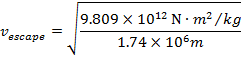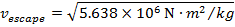2374 m/s

The escape velocity from the moon is 2374 m/s, or approximately 2.37 km/s.

#### 1 Comment on Escape Velocity Formula

1.ปั้มไลค์ // August 9, 2019 at 3:23 AM //

Like!! I blog quite often and I genuinely thank you for your information. The article has truly peaked my interest.